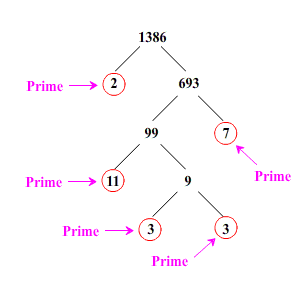# Unique Prime Factorization

The Fundamental Theorem of Arithmetic states that every natural number greater than $1$ can be written as a product of prime numbers , and that up to rearrangement of the factors, this product is unique . This is called the prime factorization of the number.

Example:

$36$ can be written as $6×6$ , or $4×9$ , or $3×12$ , or $2×18$ . But there is only one way to write it as a product where all the factors are primes:

$36=2×2×3×3$

This is the prime factorization of $36$ , often written with exponents:

$36={2}^{2}×{3}^{2}$

For a prime number such as $13$ or $11$ , the prime factorization is simply itself. Any composite number (that is, a whole number with more than two factors) has a non-trivial prime factorization.

The prime factorization of a number can be found using a factor tree . Start by finding two factors which, multiplied together, give the number. Keep splitting each branch of the tree into a pair of factors until all the branches terminate in prime numbers.

Here is a factor tree for $1386$ . We start by noticing that $1386$ is even, so $2$ is a factor. Dividing by $2$ , we get $1386=2×693$ , and we proceed from there.This shows that the prime factorization of $1386$ is $2×3×3×7×11$ .

You can use prime factorizations to figure out GCF s (Greatest Common Factors), LCM s (Least Common Multiples), and the number (and sum) of divisors of $n$ .